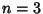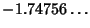## Benson's Formula

An equation for a Lattice Sum withHere, the prime denotes that summation over (0, 0, 0) is excluded. The sum is numerically equal to, a value known as the'' Madelung Constant.

Finch, S. Favorite Mathematical Constants.'' http://www.mathsoft.com/asolve/constant/mdlung/mdlung.html